Modeling a wing mast sail in heliciel Introducing the mecaflux software suite: Wings or foils modeling in heliciel Aerial propeller modeling in heliciel Boat propeller modeling in heliciel Ventilation propeller modeling in heliciel Wind turbine propeller modeling in heliciel Tidal turbine modeling in heliciel Kaplan propeller modeling in heliciel
Hydrofoil wings and sails

The objective here is to remember the basics needed to construction of a wing a hydrofoil or a propeller.(propeller can be considered as a rotary wing).We will not make, for the moment, any difference between a wing and a foil because, apart cavitation , the methode is the same.
Understand what is happening on the wing and flying, has took few centuries to humanity...and it will take some time to do it with less energy than birds.Move an anvil on 10 meters with a 10 megaton firecracker is impressive but a little expensive.

.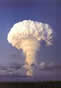The genie is to fly the heavier object possible with the least possible energy. The concept of transport speed is important because take ten days to 10 meters, even an oyster can do it.

.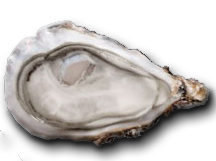Wing of quality will be one that allows us to bring the desired load at the desired speed, by applying the minimum force. To estimate the quality (efficiency) of our wing , we compare the force that it produces(The Lift) and the force that it consumes(The Drag), to the desired operating speed. efficiency =lift/drag ratio
• The lift (newtons)) is the gentle force perpendicular to the fluid stream that carries the wing that generates the thrust of the propeller. The lift is good!
• The Drag (newtons) is the nasty forces pulls the wing in the direction of fluid flow, which prevents the wing to move, the propeller to rotate. The drag is not good! This is the drag that must be compensated by providing energy to the propeller shaft to rotate.
The tests in wind tunnels or pools (numerical or experimental) give us performance coefficients (no units) that will be the the basic data for the calculation of the forces on the wings propeller blades and foils:
• The Cd = drag coefficient and
• The CL= lift coefficient of profiles.
• The lift/drag ratio gives us the quality of the profile, a high finesse can lift a heavy load with little energy..Heliciel apply by default the best lift/drag ratio angles to the blade and wings.
These coefficients are given for a wing of infinite length, this means that losses and turbulent phenomena are not taken into account. To distinguish the performance of an infinite wing (2 dimensions taken into account) , from performance of a wing of finite length (three dimensions taken into account), we talk about 2D performance for a wing of infinite length, and performance 3D for wing of finite and known length.
• The formula for the lift: Lift(2D)(en Newtons)=
CL X Wing area(m²) X Fluid density(Kg/m3) X ( fluid velocity²(m/sec) / 2).
• for the drag is the same but with the CD:Drag(2D)(en Newtons)=
CD X Wing area(m²) X Fluid density(Kg/m3) X ( fluid velocity²(m/sec)/2)
Observe that the drag forces and lift increases with the square of the speed.
The drag and lift of a propeller in the software HELICIELA wing could very well be defined by a single profile of constant width, but to answer the constraints of mechanical resistance and to obtain optimum performance, the actual shapes are generally more complex. To analyze and understand what is happening on a wing or propeller blade , it is convenient to cut it into slices, starting from the base to the tip, the shape of the sections are the profiles.The profiles describe the sectional forms of our blade. A portion flanked by two profiles is un an element of the blade or wing.
the elements and profiles of the blade
The profiles known performance that surround an item, allow us to calculate the drag and lift of the element. This method of blade elements analysis allows the calculation of propellers.This calculation using 2D performance profiles requires some corrections to take account of the length of the blade or wing which is the third dimension:
• Losses at the blade tip...or the third dimension
It is important to reiterate that the calculated drag and lift with cd and cl are theoretical values, not taking into account some losses.. Ideal wing or a blade which would not have leak, would have an efficiency equal to its 2D performance of profiles. these these drag and lift theoretical, measured in wind tunnels or calculated by numerical methods, are nominated performance 2D (2 dimensions) or "performance wing of infinite span." 2D, because it does not take into account the phenomena of the third dimension: the length of the blade or wing.
Lift resulting from the difference in pressure between the top and bottom of the wing. But the pressure differential generates leaks , because more fluid wants to get to the other side by the shortest path it finds! The shortest path is the tip of the wing or blade..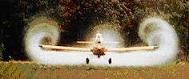Summary
• The more is the lift near the wing tip, the more are the leaks. If the lift is distributed as far from the wing tip as possible ,this will limit leakage. This is great. The wing shape which distributed lift theoretically farthest from the end is the ellipse. Some aircraft have therefore simply adopted elliptical wings. the spitfire and elliptical wings focusing lift as far as possible from wing tip to prevent leakage. In practice, a trapezoidal shape is sufficient to get an effect similar to the optimum: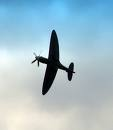• More the blade or wing is short there are more leaks. We have therefore interest to distribute the surface on a large length and small width. The concept of Aspect Ratio helps us to evaluate this distribution:
A valid formula for all forms of wing uses the surface and span:
• Aspect ratio(all forms of wing)= span (wing length)²/(wing area)
A high aspect ratio is a lift distributed on a large surface therefore a difference of pressure between lower and upper surfaces not giving too much to the fluid the desire to get to the other sideCalculate the performance of a wing considering tip vortices and tip losses requires knowing the impact these vortices on the profiles 2D performance. This can be done by calculating the deviation of the fluid induced by the vortices. Calculating the speeds induced by the vortices, we can correct angles perceived by profiles and infer the performance of the wing of finite span (3D).The calculations of these speeds is achieved by the induced vortex theory that describes the relation between the lift and the vortices. To learn more:Theory wing of finite span based on the method of distribution of vortices.
Example of the influence of the shape and aspect ratio: vortices and wingtip loss of 4 wings of shapes and different aspect ratio, shown schematically in software Heliciel
 Rectangular wing length of 2 meters Aspect ratio = 4 ratio (Lift / Drag) =16 Rectangular wing length of 9 meters Aspect ratio = 18 ratio (Lift / Drag) =32 Triangular wing length of 2 meters. Aspect ratio = 5 ratio (Lift / Drag) =19. Triangular wing length of 9 meters. Aspect ratio = 33 ratio (Lift / Drag) =36
A device reduces the blade tip leakage by placing a barrier:
• Les winglets..
• Combineaspect ratio, a good distribution of the lift and winglets is very good.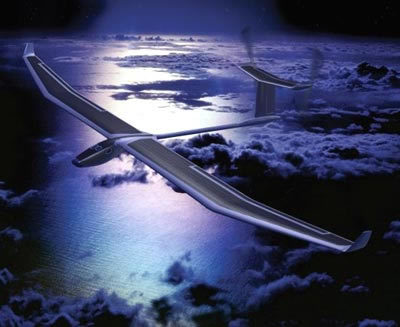• The Solar Impulse project aims to take off and fly autonomously, day and night, an airplane powered only by solar energy, to perform round the world without fuel or pollution ..
You now know the basic principles that will allow you to guide your choices when you choose your wing or blade geometry settings in HELICIEL software :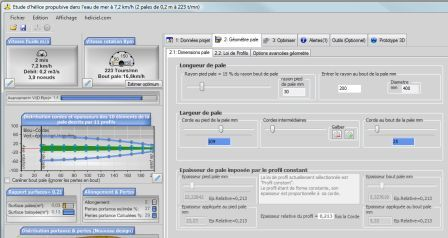We now turn to a specific phenomenon hydrofoils and marine propellers: Cavitation